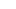# Solutions

Get detailed explanations to advanced GMAT questions.

### Question

The median length of the 13 logs in shipment x was 84 inches, and the median length of the 21 logs in shipment y was 85 inches. If the two longest logs in shipment Y were transferred to shipment X, what would be the median length, in inches, of the 15 logs in shipment X after the transfer?

I. The two logs transferred had lengths of 91 and 92 inches, respectively.

II. The longest log that was in shipment x before the transfer had length 90 inches.

Option A:

Statement (1) ALONE is sufficient, but statement (2) alone is not sufficient to answer the question asked.

Option B:

Statement (2) ALONE is sufficient, but statement (1) alone is not sufficient to answer the question asked.

Option C:

BOTH statements (1) and (2) TOGETHER are sufficient to answer the question asked, but NEITHER statement ALONE is sufficient to answer the question asked.

Option D:

Option E:

Statements (1) and (2) TOGETHER are NOT sufficient to answer the question asked, and additional data specific to the problem are needed.

Hard

### Solution

Option E is the correct answer.

### Option Analysis

The question tells us that the median of the 13 logs in shipment x is 84 inches. This implies that the length of the seventh log, when all logs are arranged in ascending order, is 84.

The question also tells us that the median of the 21 logs in shipment y is 85 inches. This implies that the length of the 12th log, when all logs are arranged in ascending order of length, is 85 inches.

Now, two longest logs are shifted from shipment y to shipment x. We need to find the median length of the 15 logs in shipment x.

Statement I is insufficient: This tells is the length of the longest two logs transferred. However, to find the median of the fifth log, we need to know the length of the 8th log which must be greater than 84 inches. However, we cannot be sure of its length.

So insufficient.

Statement II is insufficient: This tells us that the longest log is shipment x has a length of 90 inches. However, this does not tell us the lengths of the logs longer than 84 inches. So insufficient.

Together, the statements still do not tell us anything about the length of the 8th log in shipment X so still insufficient.

#### 2 thoughts on “The median length of the 13 logs in shipment x was 84 inches, and the median length of the 21 logs in shipment…”

1.Mridula says:

The explanation is not understood by me. Can you please elaborate on this.

2.abcdddddd says:

In the explanation –
“The question also tells us that the median of the 21 logs in shipment y is 85 inches. This implies that the length of the 12th log”

However, the median of 21 logs will be (21+1)/2th log which is 22/2 = 11th log (NOT 12th log). Kindly rectify this mistake.